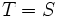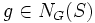# Alperin's fusion theorem in terms of conjugation families

(diff) ← Older revision | Latest revision (diff) | Newer revision → (diff)

## History

This statement was formulated and proved by Jonathan Lazare Alperin, in 1965.

## Statement

### General form

Suppose$G$ is a finite group,$S$ is a$p$-Sylow subgroup for a prime$p$ Suppose$\mathcal{F}$ is a collection of subgroups of$S$ with the property that for any subgroup$T$ of$S$, there exists$U \in \mathcal{F}$ such that:

•$U$ and$T$ are conjugate subgroups inside$G$
•$N_S(U)$ is a$p$-Sylow subgroup of$N_G(U)$

Then$\mathcal{F}$ is a conjugation family for$S$ in$G$.

### More specific form

This form states:

Let$\mathcal{F}$ be the collection of all subgroups of$S$ whose normalizer in$S$ is a Sylow subgroup of the normalizer in$G$. Then,$\mathcal{F}$ is a conjugation family for$S$ in$G$.

This follows from the more general form, and the fact that every p-subgroup is conjugate to a p-subgroup whose normalizer in the Sylow is Sylow in its normalizer.

## Proof

### Key idea: induction on index

We prove the result by inducting on the index of the subgroup$\langle A \rangle$ in$S$. Suppose$T = \langle A \rangle$ and$V = \langle B \rangle$.

### Base case for induction

The base case of induction is when$T = S$. By the conditions,$\mathcal{F}$ contains a conjugate of$S$, so$S \in \mathcal{F}$. Clearly, then$g \in N_G(S)$, so we can set$n = 1$, and$g_1 = g$.

### Induction step

The key thing to remember for the induction step is that if$T$ is a proper subgroup of$S$, then$T$ is a proper subgroup of$N_S(T)$ (and similarly for$V$). Thus, this step reduces to three parts:

• Go from$T$ to$N_S(T)$
• Use the induction to argue that we can go from$N_S(T)$ to$N_S(U)$
• Go from$N_S(V)$ back down to$V$